Mean, median and mode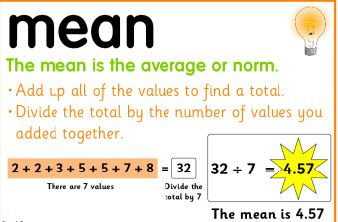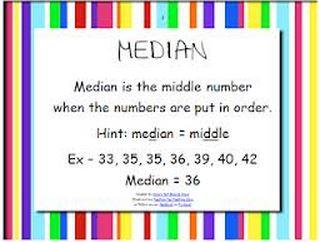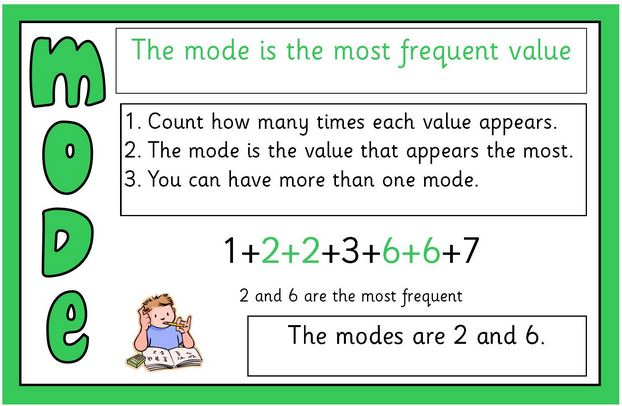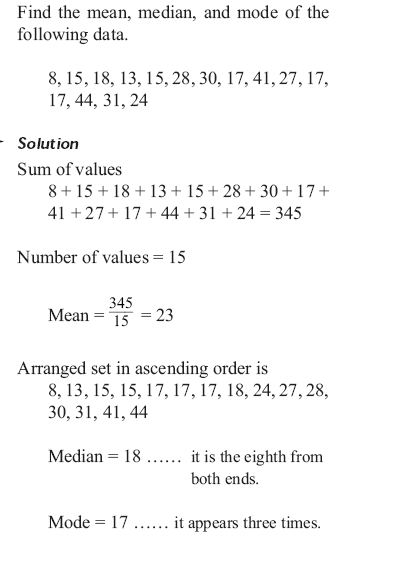#### Example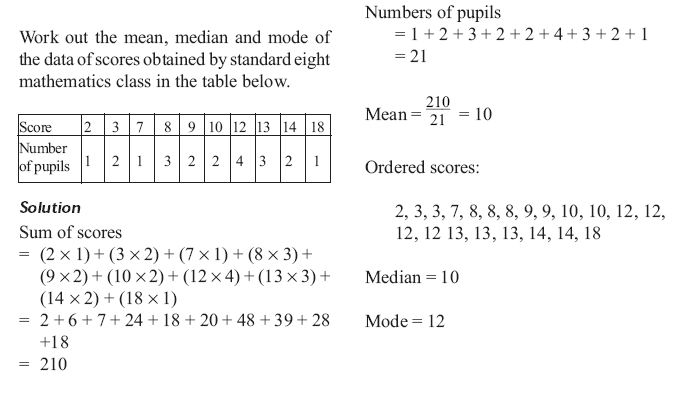### Frequency and frequency tables

The frequency of a particular data value is the number of times the data value occurs.

For example, if 4 students have a score of 80 in mathematics and then the score of 80 is said to have a frequency of 4. The frequency of a data value is often represented by f.

A frequency table is constructed by arranging collected data values in ascending order of magnitude with their corresponding frequencies.

#### Example

The marks awarded for an assignment set for a Year 8 class of 20 students were as follows:
6     7     5     7     7     8     7     6     9     7
4     10   6     8     8     9     5     6     4     8

Present this information in a frequency table.

#### Solution

To construct a frequency table, we proceed as follows:

Step 1

Construct a table with three columns.  The first column shows what is being arranged in ascending order (i.e. the marks).  The lowest mark is 4.  So, start from 4 in the first column as shown below.

 Mark Tally Frequency 4 5 6 7 8 9 10

Step 2

Go through the list of marks.  The first mark in the list is 6, so put a tally mark against 6 in the second column.

The second mark in the list is 7, so put a tally mark against 7 in the second column.

The third mark in the list is 5, so put a tally mark against 5 in the third column as shown below.

 Mark Tally Frequency 4 5 / 6 / 7 / 8 9 10

We continue this process until all marks in the list are tallied.

Step 3

Count the number of tally marks for each mark and write it in third column.

The finished frequency table is as follows: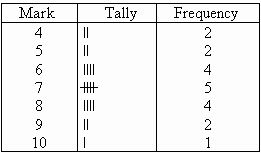### Find the mean, median mode and range for the following list of values

13, 18, 13, 14, 13, 16, 21, 13

The mean is usually average, so:

(13 + 18 + 13 + 14 +13 + 14 + 13 + 16 + 21 + 13)/ 9 =15

Note that the mean isn't a value from the original list. This is a common result. You should not assume that your mean will be one of your original numbers.

The median is the middle value, so I'll have to rewrite the list in order.

13, 13, 13, 13, 14, 14, 16, 18, 21

There are nine numbers in the list, so the middle one will be

(9 + 1) + 2 = 10/2 =5th number.

13, 13, 13, 13, 14, 14, 16, 18, 21

So the median is 14.

The mode is the number that is repeated more often than any other number, so 13 is the mode.

The largest value is 21, and the smallest is 13, so the range is 21 -13 = 8

## KCPE Topical Questions

### The Mean

1. The mean age of Mbaya, Bogi, Khadija and Hussein is 11 years. Bogi is twice as old as Mbaya. Khadija and Hussein are each 1o years old. How old is Bogi?

A. 16 years     B. 24 years     C. 20 years     D. 8 years

2. The mean weight of 6 girls is 30 kg. The total weight of 4 of the girls is 128 kg. If the remaining 2 girls weigh the same, what us the weight of each of the two girls?

A. 26       B. 30       C. 52        D. 32

3. Atieno was born when her father was 27 years old and her mother 24 years. After how many years will the mean age of Atieno and her parents be 30 years?

A. 13          B. 17         C. 39           D. 3

4. Mutiso delivered milk to a dairy in the mornings and in the afternoons. During the month of March, he delivered an average of 78 litres every morning. If he delivered a total of 3813 litres, what was the average number of litres he delivered every afternoon?

A. 45          B. 49           C. 123         D. 1395

5. Ten pupils in a class sat for a test and had a mean score of 6.3. the scores of nine if the pupils were 3, 4, 5, 6, 7, 8, 8, 8 and9. What was the score of the tenth pupil?

A. 5        B. 7           C. 8         D. 10

6. The table below shows the number of pupils present in a class in one week.

 Days of the week Mon Tue Wed Thu Fri Number of pupils 41 45 43 45 36

What was the mean attendance of the class that week?

A. 45        B. 43       C. 42       D. 40

7. A farmer sold milk for all the days of the week. The table below shows the amount of milk the farmer sold for six of the seven days.

 Day Mon Tue Wed Thu Fri Sat Sun Amount in Kg 410 315 400 410 300 420

One kilogram of milk was sold at sh 18. If the mean sale per day was 380 kg, how much more money did the farmer get from selling the milk on Friday than on Tuesday?

A. Sh 7290      B. Sh 5670      C. Sh 1620       D. Sh 1170

8. In a class of 50 pupils, the mean score in an English test is 60. The mean score for the last 40 pupils was 55. What was the score for the first 10 pupils?

A. 600          B. 800       C. 2200        D. 3000

### Mode

1. The mean age of 3 Standard VIII pupils is 14 years. Which one of the following is the mode of their ages if one of the pupils is 12 years old?

A. 17         B. 14        C. 16         D. 15

2. A shoe dealer recorded the sizes of the first ten pairs of shoes sold as follows:

6  10  7  9  10  11  7  6  7  8

What was the mode of these shoe sizes?

A. 11        B. 8.3           C. 7.5           D. 7

### Median

1. Seven pupils sat for a test. Three of them scored 42 marks each. The other four scored 53, 55, 50 and 45 marks. What was the median score?

A. 42      B. 45      C. 47       D. 50

2. The mean of six numbers is 5.5. Five of these numbers are 2, 4, 8, 4 and 10. What is the median of the six numbers?

A. 4       B. 4.5     C. 5           D. 5.5

3. In one week Mwai’s shoe shop was opened from Monday to Saturday. In the first three days he sold 16 pairs of shoes that day. On the fourth day, he sold 22 pairs and in the last two days, he sold 25 pairs each day. What was the median of the numbers of shoes sold that week?

A. 16       B. 20        C. 19        D. 22

4. Ten pupils obtained the marks recorded below:

3  3  5  7  8  5  8  5  6  9

What was the median mark?

A. 5         B. 5.5       B. 5.9          D. 6.5

5. The mean weight of eight pupils is 37.5 kg. Six of the pupils weigh 30 kg, 39 kg, 34 kg, 35 kg, 40 kg and 38 kg. If the remaining two pupils had equal weights, what is the median weight of the eight pupils?

A. 38.5 kg     B. 42 kg      C. 38 kg      D. 36.5 kg

6. The table below represents marks scored by Adong’a in a test.

 SUBJECT MATHS B.Ed. GHC MUSIC ART/ CRAFT KISW ENG SCIE CRE Score out of 50 38 40 23 25 28 35 41 40 36

What was her median score?

A. 28       B. 34      C. 36      D. 40